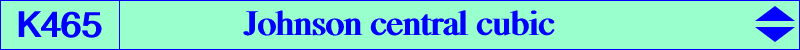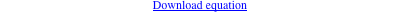too complicated to be written here. Click on the link to download a text file.X(3), X(4), X(5), X(1154), X(1157), X(1263), X(14072), X(14979), X(14980) Qa, Qb, Qc : reflections of A, B, C in X(5) i.e. centers of the Johnson circles P = X(14979) = X(3)X(1291) /\ X(5)X(476) /\ X(30)X(930), etc, on the circumcircle Q = X(14980) = reflection of P in X(5), on the circle QaQbQc.The cubics K026 and K044 contain the centers of the three Johnson circles. See a definition here. They generate a pencil of central cubics with center X(5), the nine point center. This pencil contains one and only one circular cubic which is K465. Thus, K465 is a central focal cubic with center and singular focus X(5). See Table 76. The isogonal transform of K465 is K466. Locus properties • locus of M such that the nine-points circles of MAQa, MBQb, MCQc are coaxial, together with the line at infinity (see Hyacinthos #27292). • locus of pivots of circular pKs whose orthic line passes through X(195). • locus of isopivots of circular pKs passing through X(5). The locus of pivots is K1350. See K050, K060, K067.